9 篇文章 0 订阅

# GJK 算法(Gilbert–Johnson–Keerthi)

GJK与SAT一样，仅在凸包上运行。GJK更具吸引力的功能之一是，它可以支持实现“
support ”函数的任何形状（我们将在后面讨论）。因此，与SAT不同，您不需要增加特殊的代码或算法来处理弯曲的形状。

GJK是一种迭代方法，但是收敛速度非常快，如果使用最终的穿透/分离向量进行约束，则可以在几乎恒定的时间内运行。在3D环境中，它是SAT的更好替代方案，因为SAT必须测试轴数。

GJK的初衷是确定两个凸包之间的距离。GJK还可以用于在小距离穿透情况下获取碰撞信息，并可以通过其他算法进行补充以实现更大距离的穿透。

GJK算法在很大程度上依赖于称为闵科夫斯基和的概念。闵科夫斯基和在概念上非常容易理解。假设您有两个形状，这些形状的闵科夫斯基和就是shape1中的所有点加到shape2中的所有点上{得到的是另外一个更大更复杂的形状}：

A + B = {a + b |a∈A，b∈B}

A – B = {a – b |a∈A，b∈B}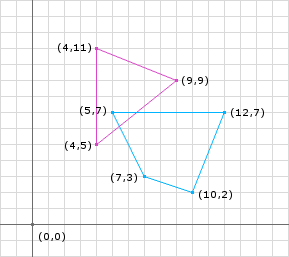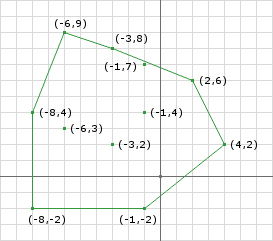Support函数

 1 2 3 4 5 6 7 8 9 10 public Point support(Shape shape1, Shape shape2, Vector d) {   // d is a vector direction (doesn't have to be normalized)   // get points on the edge of the shapes in opposite directions   Point p1 = shape1.getFarthestPointInDirection(d);   Point p2 = shape2.getFarthestPointInDirection(d.negative());   // perform the Minkowski Difference   Point p3 = p1.subtract(p2);   // p3 is now a point in Minkowski space on the edge of the Minkowski Difference   return p3; }

 1 2 3 p1 = (9, 9); p2 = (5, 7); p3 = p1 - p2 = (4, 2);

 1 2 3 p1 = (4, 5); p2 = (12, 7); p3 = p1 - p2 = (-8, -2);

 1 2 3 p1 = (4, 11); p2 = (10, 2); p3 = p1 - p2 = (-6, 9);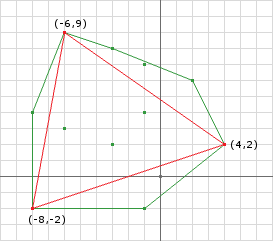1 2 3 p1 = (4, 5); p2 = (5, 7); p3 = p1 - p2 = (-1, -2);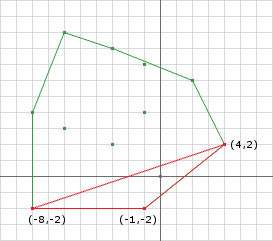1 2 3 4 5 6 7 8 d = ... a = support(..., d) d = ... b = support(..., d) AB = b - a AO = ORIGIN - a d = (AB x AO) x AB c = support(..., d)

 1 2 3 4 5 6 7 d = ... a = support(..., d) b = support(..., -d) AB = b - a AO = ORIGIN - a d = (AB x AO) x AB c = support(..., d)

 1 2 3 4 5 6 7 8 9 10 11 12 13 14 15 16 17 18 19 20 21 22 23 24 25 26 27 28 29 Vector d = // choose a search direction // get the first Minkowski Difference point Simplex.add(support(A, B, d)); // negate d for the next point d.negate(); // start looping while (true) {   // add a new point to the simplex because we haven't terminated yet   Simplex.add(support(A, B, d));   // make sure that the last point we added actually passed the origin   if (Simplex.getLast().dot(d) <= 0) {     // if the point added last was not past the origin in the direction of d     // then the Minkowski Sum cannot possibly contain the origin since     // the last point added is on the edge of the Minkowski Difference     return false;   } else {     // otherwise we need to determine if the origin is in     // the current simplex     if (Simplex.contains(ORIGIN)) {       // if it does then we know there is a collision       return true;     } else {       // otherwise we cannot be certain so find the edge who is       // closest to the origin and use its normal (in the direction       // of the origin) as the new d and continue the loop       d = getDirection(Simplex);     }   } }

 1 2 3 4 d = c2 - c1 = (9, 5) - (5.5, 8.5) = (3.5, -3.5) = (1, -1); p1 = support(A, B, d) = (9, 9) - (5, 7) = (4, 2); Simplex.add(p1); d.negate() = (-1, 1);

（A x B）x C = B（C.dot（A））– A（C.dot（B））来评估三乘积。

 1 2 3 4 5 6 7 8 9 10 11 last = support(A, B, d) = (4, 11) - (10, 2) = (-6, 9); proj = (-6, 9).dot(-1, 1) = 6 + 9 = 15 // we past the origin so check if we contain the origin // we dont because we are line // get the new direction by (AB x AO) x AB AB = (-6, 9) - (4, 2)  = (-10, 7); AO = (0, 0) - (-6, 9) = (6, -9); (AB x AO) x AB = AO(149) - AB(-123)                = (894, -1341) - (1230, -861)                = (-336, -480)                = (-0.573, -0.819)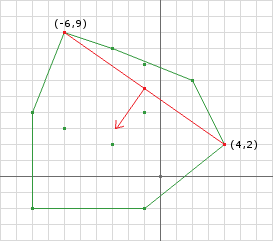1 2 3 4 5 6 7 8 9 10 11 12 last = support(A, B, d) = (4, 5) - (12, 7) = (-8, -2) proj = (-8, -2).dot(-336, -480) = 2688 + 960 = 3648 // we past the origin so check if we contain the origin // we dont (see Figure 6a) // the new direction will be the perp of (4, 2) and (-8, -2) // and the point (-6, 9) can be removed AB = (-8, -2) - (4, 2)  = (-12, -4); AO = (0, 0) - (-8, -2) = (8, 2); (AB x AO) x AB = AO(160) - AB(-104)                = (1280, 320) - (1248, 416)                = (32, -96)                = (0.316, -0.948)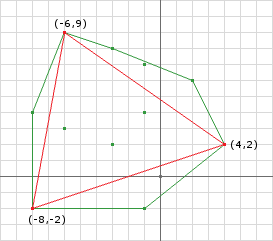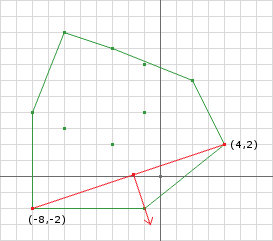1 2 3 4 last = support(A, B, d) = (4, 5) - (5, 7) = (-1, -2) proj = (-1, -2).dot(32, -96) = -32 + 192 = 160 // we past the origin so check if we contain the origin // we do (Figure 7)!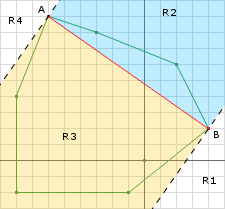1 2 3 4 // the perp of AB in the direction of O can be found by AB = B - A; AO = O - A; perp = (AB x AO) x AB;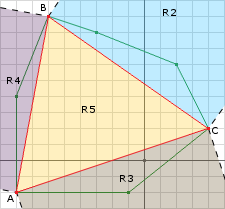1 2 3 4 5 6 7 8 9 10 11 AB = (-6, 9) - (-8, -2) = (2, 11) AC = (4, 2) - (-8, -2) = (12, 4) // (AC x AB) x AB = AB(AB.dot(AC)) - AC(AB.dot(AB)) ABPerp = AB(68) - AC(125)        = (136, 748) - (1500, 500)        = (-1364, 248)        = (-11, 2) // compute AO AO = (0, 0) - (-8, -2) = (8, 2) ABPerp.dot(AO) = -11 * 8 + 2 * 2 = -84 // its negative so the origin does not lie in R4

 1 2 3 4 5 6 7 8 9 10 11 AB = (-6, 9) - (-8, -2) = (2, 11) AC = (4, 2) - (-8, -2) = (12, 4) // (AB x AC) x AC = AC(AC.dot(AB)) - AB(AC.dot(AC)) ACPerp = AC(68) - AB(160)        = (816, 272) - (320, 1760)        = (496, -1488)        = (1, -3) // compute AO AO = (0, 0) - (-8, -2) = (8, 2) ACPerp.dot(AO) = 1 * 8 + -3 * 2 = 2 // its positive so that means the origin lies in R3

 1 (AC x AO) x AC

 1 2 3 4 5 6 7 8 9 10 11 12 13 14 15 16 17 18 19 20 21 22 23 24 25 26 27 28 29 30 31 32 33 34 35 36 37 38 39 40 41 42 43 44 45 46 47 48 49 50 51 52 53 54 55 56 57 58 59 60 61 62 63 64 65 66 67 68 69 70 71 Vector d = // choose a search direction // get the first Minkowski Difference point Simplex.add(support(A, B, d)); // negate d for the next point d.negate(); // start looping while (true) {   // add a new point to the simplex because we haven't terminated yet   Simplex.add(support(A, B, d));   // make sure that the last point we added actually passed the origin   if (Simplex.getLast().dot(d) <= 0) {     // if the point added last was not past the origin in the direction of d     // then the Minkowski Sum cannot possibly contain the origin since     // the last point added is on the edge of the Minkowski Difference     return false;   } else {     // otherwise we need to determine if the origin is in     // the current simplex     if (containsOrigin(Simplex, d) {       // if it does then we know there is a collision       return true;     }   } }   public boolean containsOrigin(Simplex s, Vector d) {   // get the last point added to the simplex   a = Simplex.getLast();   // compute AO (same thing as -A)   ao = a.negate();   if (Simplex.points.size() == 3) {     // then its the triangle case     // get b and c     b = Simplex.getB();     c = Simplex.getC();     // compute the edges     ab = b - a;     ac = c - a;     // compute the normals     abPerp = tripleProduct(ac, ab, ab);     acPerp = tripleProduct(ab, ac, ac);     // is the origin in R4     if (abPerp.dot(ao) > 0) {       // remove point c       Simplex.remove(c);       // set the new direction to abPerp       d.set(abPerp);     } else {       // is the origin in R3       if (acPerp.dot(ao) > 0) {         // remove point b         Simplex.remove(b);         // set the new direction to acPerp         d.set(acPerp);       } else{         // otherwise we know its in R5 so we can return true         return true;       }     }   } else {     // then its the line segment case     b = Simplex.getB();     // compute AB     ab = b - a;     // get the perp to AB in the direction of the origin     abPerp = tripleProduct(ab, ao, ab);     // set the direction to abPerp     d.set(abPerp);   }   return false; }

02-15956908-11266
04-24
03-131241
08-30963
03-21404
04-182950
04-05
05-26
01-297136点击重新获取扫码支付余额充值